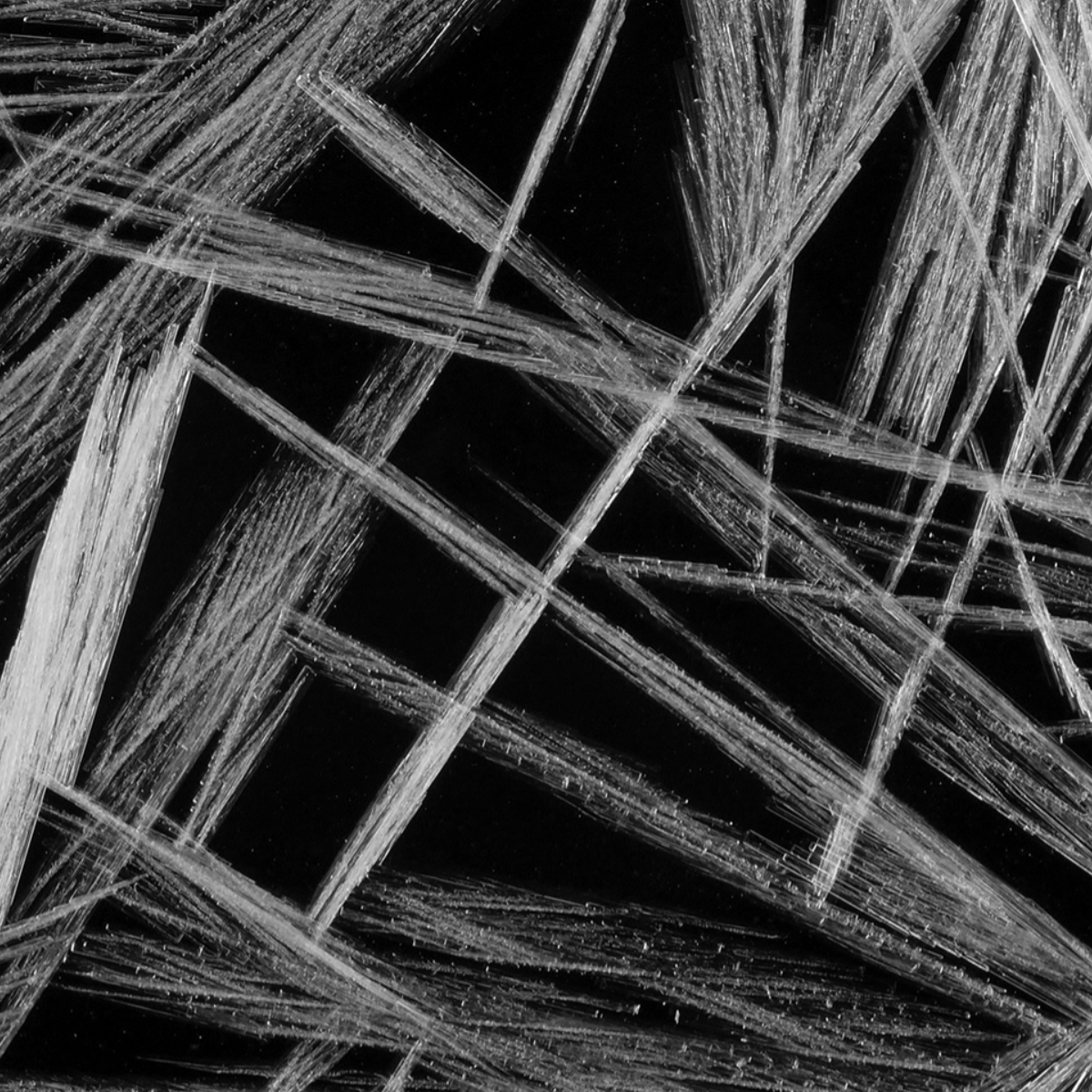# Unbiased degree-preserving randomization of directed binary networks

Unbiased randomisation processes generate sophisticated synthetic networks for modelling and testing the properties of real-world networks.

Physical Review E 85, 46103 (2012)

E. Roberts, A. CoolenRandomizing networks using a naive “accept-all” edge-swap algorithm is generally biased. Building on recent results for nondirected graphs, we construct an ergodic detailed balance Markov chain with nontrivial acceptance probabilities for directed graphs, which converges to a strictly uniform measure and is based on edge swaps that conserve all in and out degrees. The acceptance probabilities can also be generalized to define Markov chains that target any alternative desired measure on the space of directed graphs in order to generate graphs with more sophisticated topological features. This is demonstrated by defining a process tailored to the production of directed graphs with specified degree-degree correlation functions. The theory is implemented numerically and tested on synthetic and biological network examples.

## More in Intelligence of graphs

• Arxiv

### Transitions in loopy graphs

The generation of large graphs with a controllable number of short loops paves the way for building more realistic random networks.

• Journal of Economic Interaction and Coordination

### Bursting dynamic networks

A mathematical model captures the temporal and steady state behaviour of networks whose two sets of nodes either generate or destroy links.

• Journal of Physics A

### Exactly solvable random graphs

An explicit analytical solution reproduces the main features of random graph ensembles with many short cycles under strict degree constraints.

• Journal of Physics A

### Tailored random graph ensembles

New mathematical tools quantify the topological structure of large directed networks which describe how genes interact within a cell.

• Journal of Physics A

### Entropies of graph ensembles

Explicit formulae for the Shannon entropies of random graph ensembles provide measures to compare and reproduce their topological features.

• Journal of Physics A

### Spin systems on Bethe lattices

Exact equations for the thermodynamic quantities of lattices made of d-dimensional hypercubes are obtainable with the Bethe-Peierls approach.

• ESAIM: Proceedings and surveys

### Random graphs with short loops

The analysis of real networks which contain many short loops requires novel methods, because they break the assumptions of tree-like models.